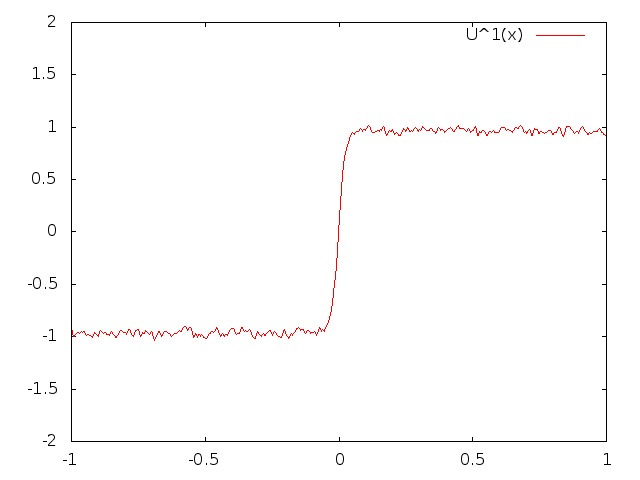# MASDOC MSc Research

### Summer Research Project

My summer research project looked at the support of diffusion processes with irregular drift coefficients, supervised by Dr. Xue-Mei Li. The Stroock-Varadhan support theorem characterises the supports of the laws of certain diffusion processes as the closure of the space of solutions to ODEs formed by replacing the Brownian motion with smoother control paths. If the drift coefficient is irregular enough these ODEs may not be well-posed. We considered regularisation of these ODEs via noise, as well as interpreting them as differential inclusions and looking at Filippov solutions.

[pdf]

### Research Study Group

I worked with Graham Hobbs, David O'Connor and Daniel Sanz-Alonso on the SPDEs project, supervised by Prof. Martin Hairer and Dr. Hendrik Weber. The project was concerned with the stochastic Allen-Cahn equation:

### $\frac{\partial u}{\partial t} = \frac{1}{2}\Delta u + f_\varepsilon(u) + \sigma\sqrt{\varepsilon}\,\xi$

where $$\xi$$ is space-time white noise, $$\sigma > 0$$ and $$f_\varepsilon$$ is the derivative of a double well potential. We looked at the sharp interface limit of the equation with asymmetric potential in one dimension, and performed some numerical simulations for the equation in two-dimensions.